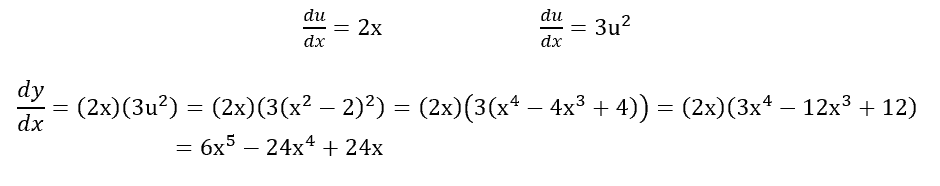# PCAT Quantitative Reasoning – Derivative of a Nested Function

Given the function f(x) = (x2 – 2)3, find f’(x).

A. 3x2 – 6
B. 3x4 – 12x3 + 12
C. 6x4 – 12
D. 6x5 – 24x4 + 24x

##### Click for Explanation

D is correct. Finding the derivative of this nested function requires us to use the Chain Rule:According to the Chain Rule, we now must find the derivative of u = x2 – 2 and y = u3 and multiply these together. Then we can plug in x2 – 2 for u and solve:#### Submit a Comment

This site uses Akismet to reduce spam. Learn how your comment data is processed.